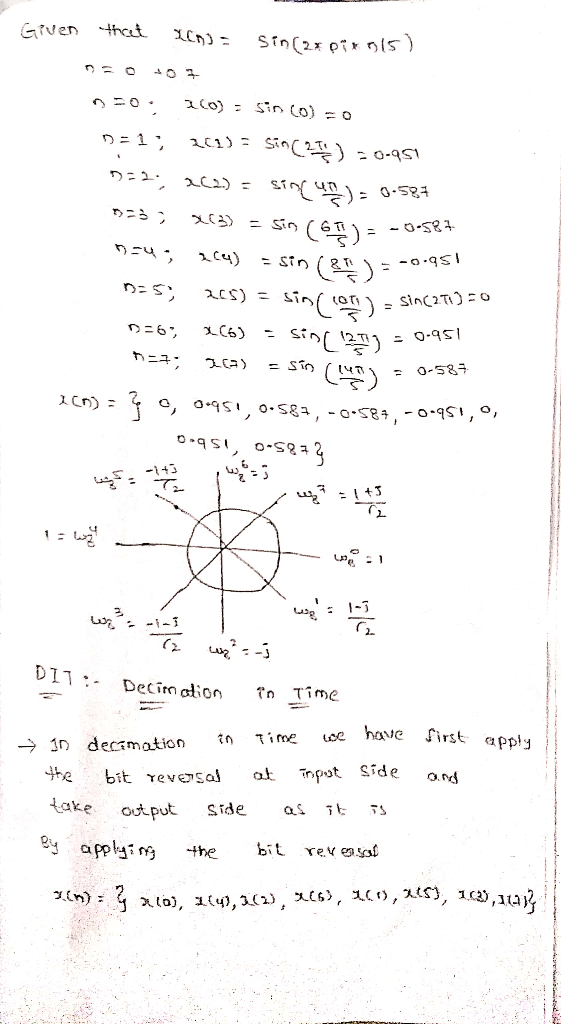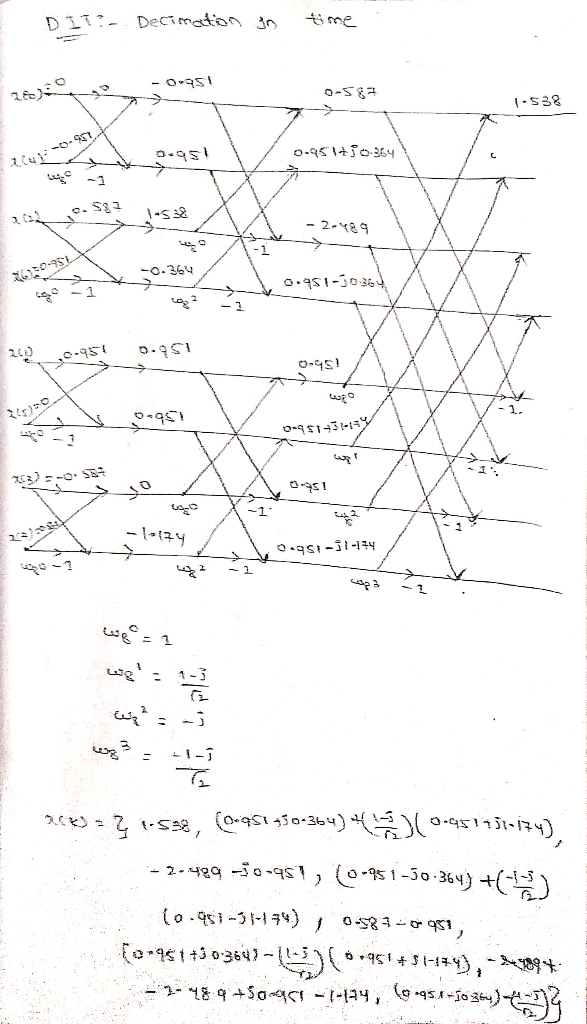##### Question

In: Electrical Engineering

# For a signal x(n)=sin(2*pi*n/5) defined for n=0to7, evaluate the Fast Fourier Transform using signal flow graph....

For a signal x(n)=sin(2*pi*n/5) defined for n=0to7, evaluate the Fast Fourier Transform using signal flow graph. (Use decimation in Time Algorithm).

## Solutions

##### Expert Solutionupvote if it is helpful to you

## Related Solutions

##### 4. For a signal x(n)=sin(2*pi*n/3) defined for n=0to7, evaluate the Fast Fourier Transform using signal flow...
4. For a signal x(n)=sin(2*pi*n/3) defined for n=0to7, evaluate the Fast Fourier Transform using signal flow graph. (Use decimation in frequency Algorithm)
##### What is the effect of the filter on the Fast Fourier Transform of voice signal? Is...
What is the effect of the filter on the Fast Fourier Transform of voice signal? Is quantization visible? How can you tell?
##### USING MATLAB.... a.) Create anonymous functions: fa(x)=sin(x^2) fb(x)=sin^2(x) b.) Evaluate them both at x=1/2 pi c.)Evaluate...
USING MATLAB.... a.) Create anonymous functions: fa(x)=sin(x^2) fb(x)=sin^2(x) b.) Evaluate them both at x=1/2 pi c.)Evaluate them both at x=(0,1,2,...,10)^T d.) Calculate fa(fb(2)) and fb(fa(2))
##### Find the Fourier series expansion of f(x)=sin(x) on [-pi,pi]. Show all work and reasoning.
Find the Fourier series expansion of f(x)=sin(x) on [-pi,pi]. Show all work and reasoning.
##### Find the real Fourier series of the piece-wise continuous periodic function f(x) = x+sin(x) -pi<=x<pi
Find the real Fourier series of the piece-wise continuous periodic function f(x) = x+sin(x) -pi<=x<pi
##### 1. is sin(pi/4) causal? 2. is sin(pi/4) stable? 3. is delta(n+1) causal? 4.  = ? 5. If...
1. is sin(pi/4) causal? 2. is sin(pi/4) stable? 3. is delta(n+1) causal? 4.  = ? 5. If function w [ n ] is convolved with , what will the result be? 6. if a system with signal length 4 is convolved with its own system response, what will the length of that signal be? 7. In an LTI system, x[n] * h[n]= y[n]. What is x[n-3] * h[n-2] =?
##### S(x) is a cubic spline for the function f(x) = sin(pi x/2) + cos(pi x/2) at...
S(x) is a cubic spline for the function f(x) = sin(pi x/2) + cos(pi x/2) at the nodes x0 = 0 , x1 = 1 , x2 = 2 and satisfies the clamped boundary conditions. Determine the coefficient of x3 in S(x) on [0,1] ans. pi/2 -3/2
##### What increase in speed can be expected in using a fast Fourier transform algorithm rather than...
What increase in speed can be expected in using a fast Fourier transform algorithm rather than direct arithmetic to compute the Fourier transform of an image of size 1024 × 1024?
##### Find the Fourier Series for the function defined over -5 < x < 5 f(x) =...
Find the Fourier Series for the function defined over -5 < x < 5 f(x) = -2 when -5<x<0 and f(x) = 3 when 0<x<5 You can use either the real or complex form but must show work. Plot on Desmos the first 10 terms of the series along with the original function.
##### Determine the Fourier transform of a Gaussian pulse defined as x(t) = e?t2. Plot both x(t) and X(?).
Determine the Fourier transform of a Gaussian pulse defined as x(t) = e?t2. Plot both x(t) and X(?).